# laneMarkingVertices

Lane marking vertices and faces in driving scenario

## Syntax

``[lmv,lmf] = laneMarkingVertices(scenario)``
``[lmv,lmf] = laneMarkingVertices(ac)``

## Description

example

````[lmv,lmf] = laneMarkingVertices(scenario)` returns the lane marking vertices, `lmv`, and lane marking faces, `lmf`, contained in driving scenario `scenario`. The `lmf` and `lmv` outputs are in the world coordinates of `scenario`. Use lane marking vertices and faces to display lane markings using the `laneMarkingPlotter` function with a bird's-eye plot.```

example

````[lmv,lmf] = laneMarkingVertices(ac)` returns lane marking vertices and faces in the coordinates of driving scenario actor `ac`.```

## Examples

collapse all

Create a driving scenario containing a car and pedestrian on a straight road. Then, create and display the lane markings of the road on a bird's-eye plot.

Create an empty driving scenario.

```scenario = drivingScenario; ```

Create a straight, 25-meter road segment with two travel lanes in one direction.

```lm = [laneMarking('Solid') laneMarking('Dashed','Length',2,'Space',4) laneMarking('Solid')]; l = lanespec(2,'Marking',lm); road(scenario,[0 0 0; 25 0 0],'Lanes',l); ```

Add to the driving scenario a pedestrian crossing the road at 1 meter per second and a car following the road at 10 meters per second.

```ped = actor(scenario,'ClassID',4,'Length',0.2,'Width',0.4,'Height',1.7); car = vehicle(scenario,'ClassID',1); smoothTrajectory(ped,[15 -3 0; 15 3 0],1); smoothTrajectory(car,[car.RearOverhang 0 0; 25-car.Length+car.RearOverhang 0 0],10); ```

Display the scenario and corresponding chase plot.

```plot(scenario) ```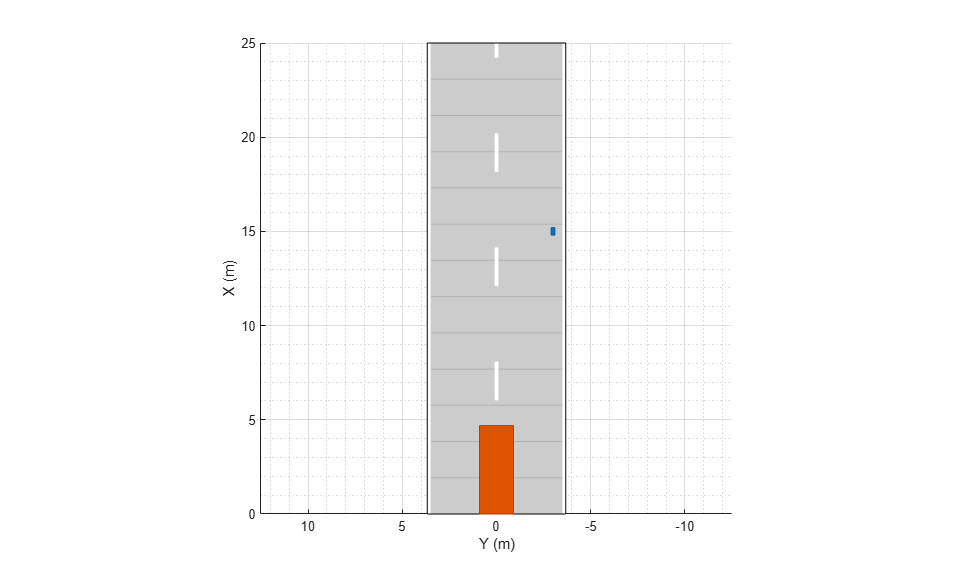```chasePlot(car) ```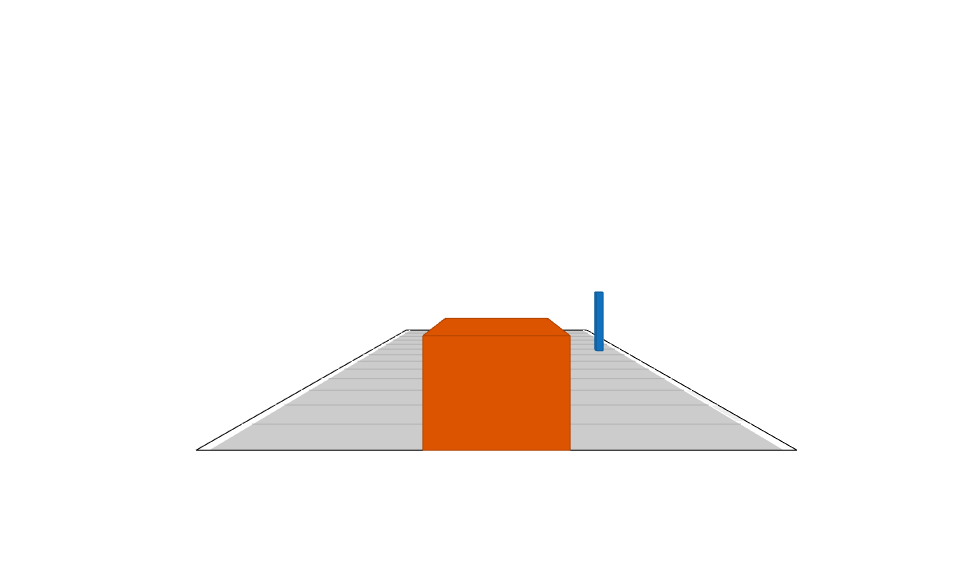Run the simulation.

1. Create a bird's-eye plot.

2. Create an outline plotter, lane boundary plotter, and lane marking plotter for the bird's-eye plot.

3. Obtain the road boundaries and target outlines.

4. Obtain the lane marking vertices and faces.

5. Display the lane boundaries and lane markers.

6. Run the simulation loop.

```bep = birdsEyePlot('XLim',[-25 25],'YLim',[-10 10]); olPlotter = outlinePlotter(bep); lbPlotter = laneBoundaryPlotter(bep); lmPlotter = laneMarkingPlotter(bep,'DisplayName','Lanes'); legend('off'); while advance(scenario) rb = roadBoundaries(car); [position,yaw,length,width,originOffset,color] = targetOutlines(car); [lmv,lmf] = laneMarkingVertices(car); plotLaneBoundary(lbPlotter,rb); plotLaneMarking(lmPlotter,lmv,lmf); plotOutline(olPlotter,position,yaw,length,width, ... 'OriginOffset',originOffset,'Color',color); end ```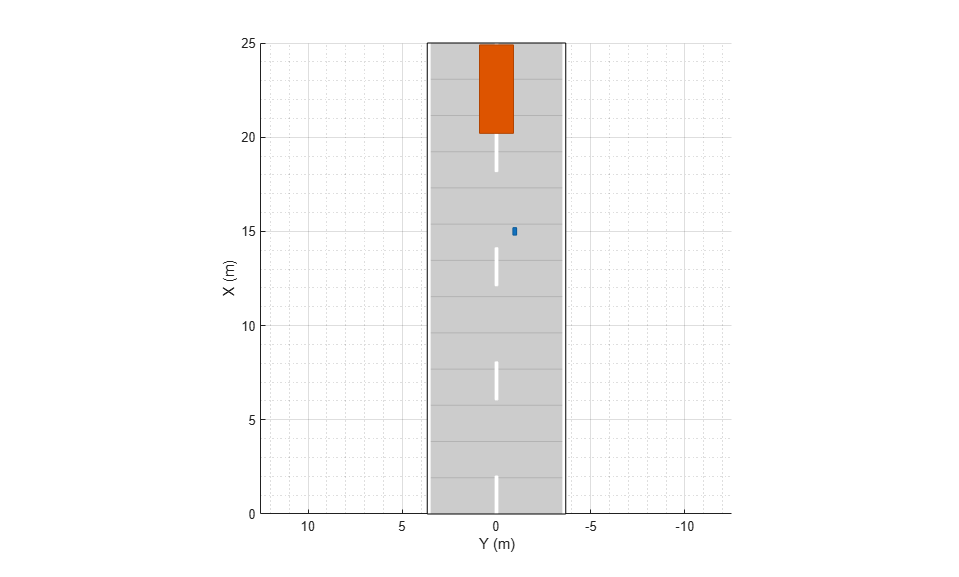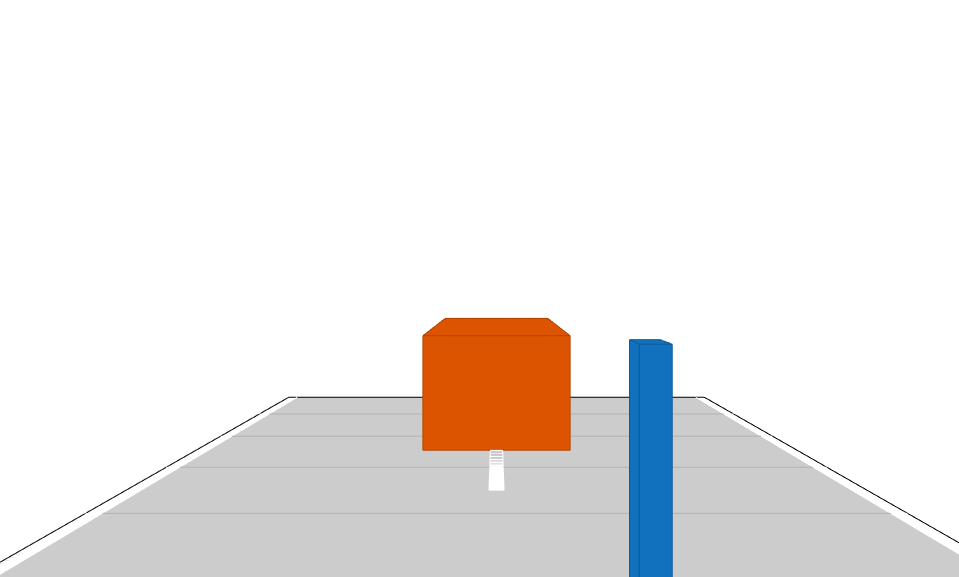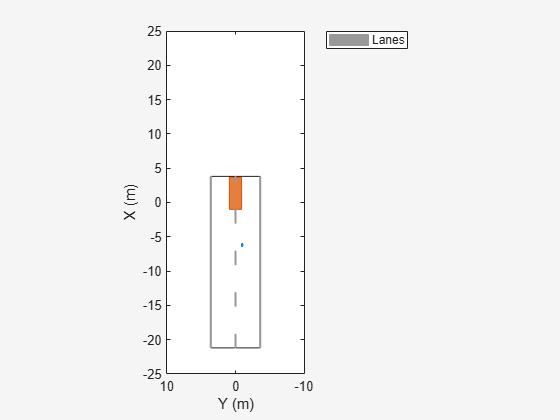## Input Arguments

collapse all

Driving scenario, specified as a `drivingScenario` object.

Actor belonging to a `drivingScenario` object, specified as an `Actor` or `Vehicle` object. To create these objects, use the `actor` and `vehicle` functions, respectively.

## Output Arguments

collapse all

Lane marking vertices, returned as a real-valued matrix. Each row of the matrix represents the (x, y, z) coordinates of a vertex.

Lane marking faces, returned as a integer-valued matrix. Each row of the matrix contains the vertex connections that define a face for one lane marking. For more details, see Faces.

## Algorithms

This function uses the `patch` function to define lane marking vertices and faces.

Introduced in R2018a